# Universal Set

Universal Set
Go back to  'Sets'

In everyday life, we come across different groups of objects like a pack of cards, a cricket team or a crowd of people, etc.

In this lesson, we will learn about universal sets.

All the stars in a milky way galaxy is a good example of a universal set, if we talk about all the stars in the milky way galaxy.This type of universal set is appropriate for astronomers.

When we study numbers in mathematics, we are interested in the set of natural numbers.

This basic set is a universal set and its subsets are even numbers, prime numbers, etc.

## What Is Meant By Universal Set?

The universal set is the set of all elements or members of all related sets.

For example, in human population studies, the universal set is the set of all the people in the world.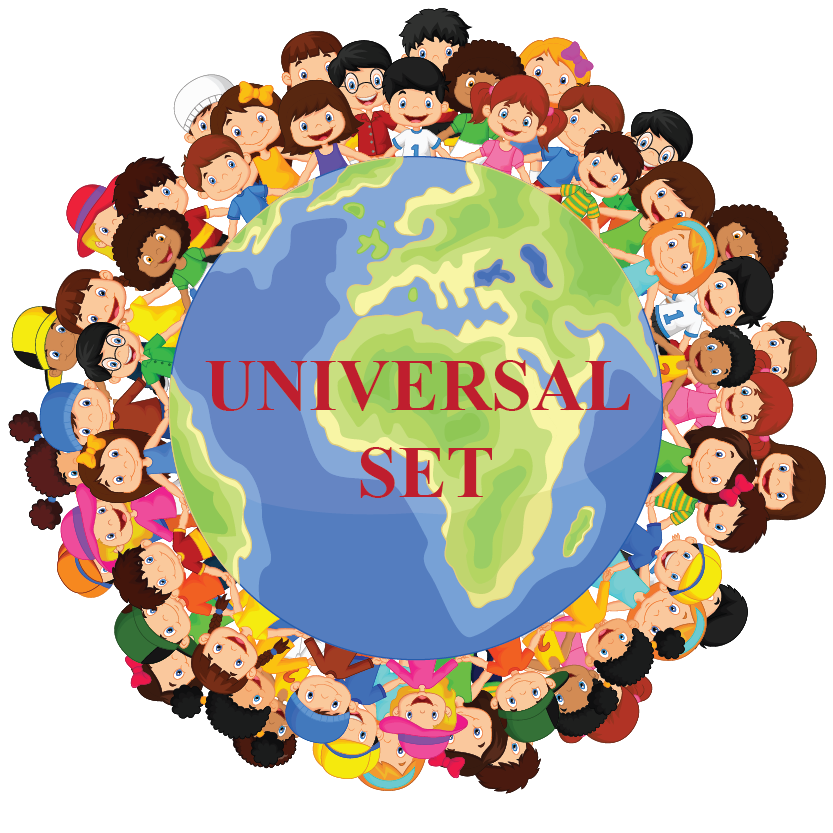We hope you understood that a universal set is a collection of all the elements relevant to a particular topic.

### Universal Set Is Finite Or Infinite Set

A universal set can be either a finite or infinite set.

The set of natural numbers is a typical example of an infinite universal set.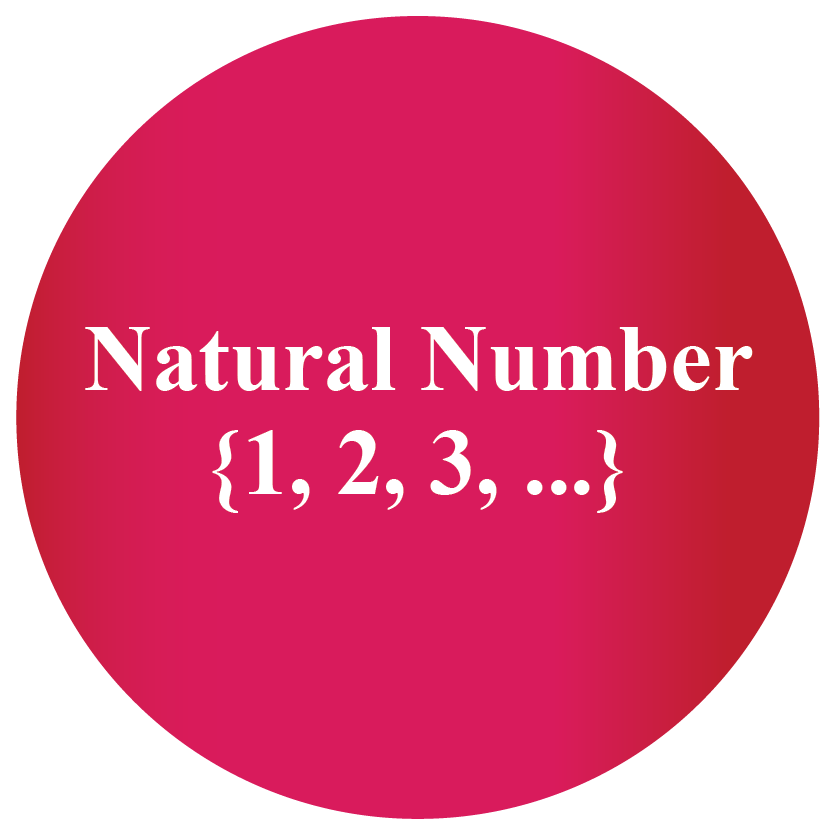The ellipsis mark (...) denotes that the set goes on without an end.

Can you guess an example of a finite universal set?

The set of all the presidents of the United States is a good example of a universal set that is finite.## Notation For Universal Set

We usually use the capital letter "U" to denote a universal set.Most of the time we use the Venn diagram to show the relationship between sets.

Venn diagrams are the graphical representation of the sets.

The universal set is represented by rectangles and its subsets are represented by circles.

Look at the Venn diagram shown below.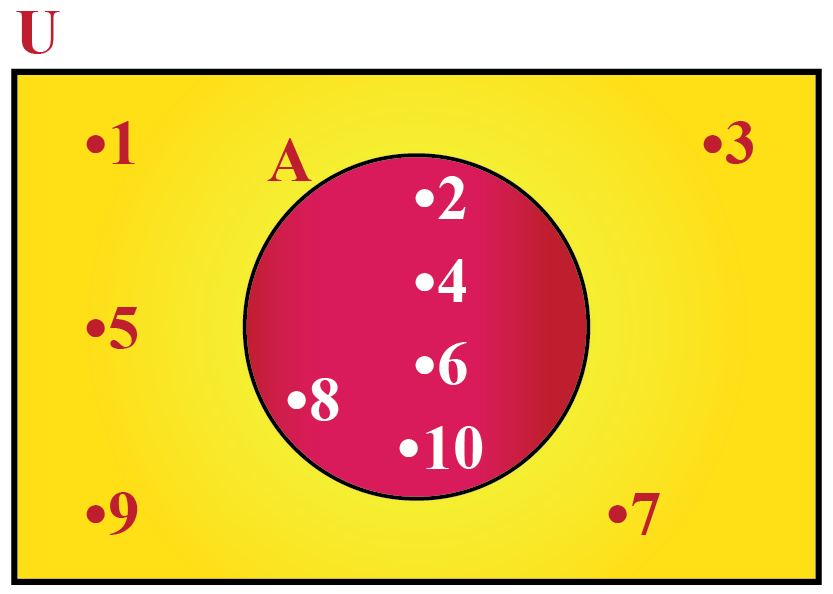Here, U = {1, 2, 3, ..., 10} is the universal set of which A = {2, 4, 6, 8, 10} is a subset.

## Venn Diagram Calculator

The simulation below shows the Venn diagram for the chosen set relations.

Choose the relation between the sets for which you want to see the Venn diagram.

## Solved Examples

 Example 1

Consider the sets, U = {1, 2, 3, 4, 5, 6, 7, 8, 9}, A = {3, 7, 9} and B = {4, 8}

Can you draw a Venn diagram to represent these sets?

### Solution

Since sets A and B are the subsets of the universal set U, we put these two sets in a circle.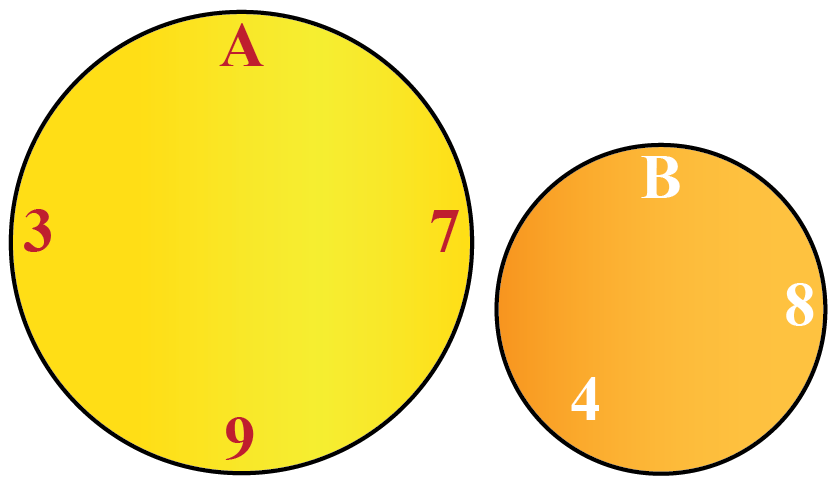Clearly, A and B are disjoint sets because they have no common element.

All the elements that are not contained in A and B are contained in the universal set.

The Venn diagram is shown below.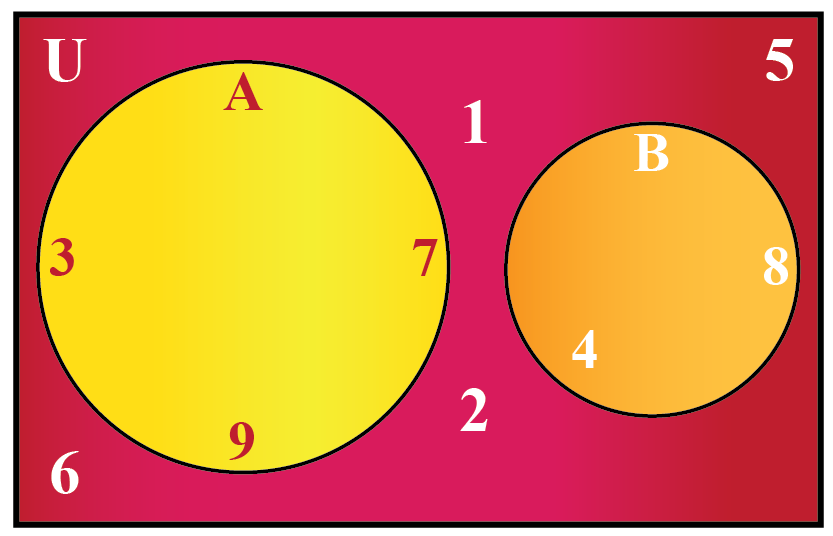So, the required Venn diagram is shown above.
 Example 2

Let U be the set of all whole numbers less than 12, P be the set of prime numbers and Q be the set of even numbers.

Can you draw a Venn diagram to represent these sets?

Solution

The given sets can be written as:

U = {0, 1, 2, 3, 4, 5, 6, 7, 8, 9, 10, 11}

P = {2, 3, 5, 7, 11}

Q = {0, 2, 4, 6, 8, 10}

The Venn diagram is shown below.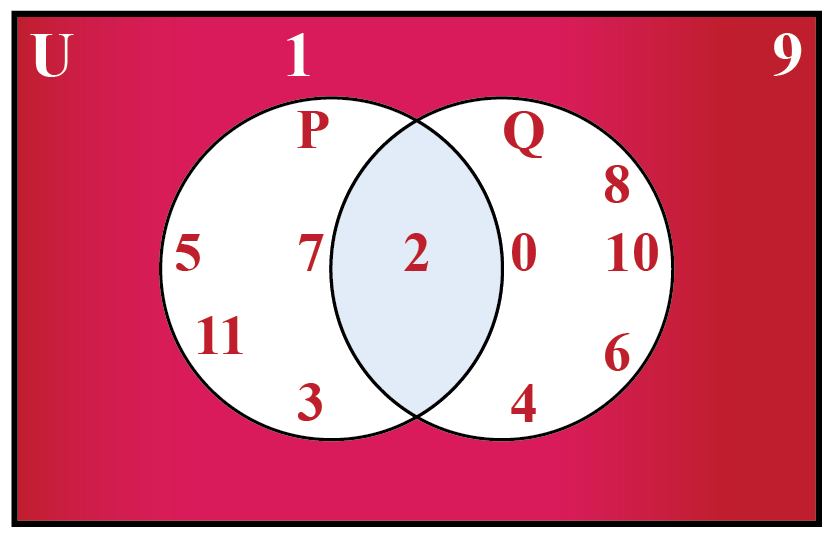So, the required Venn diagram is shown above.
 Example 3

Consider the universal set U = {2, 4, 5, 14, 17, 28, 35, 52}

List the elements of the following sets:

i) $$A={x: x \text{ is a factor of 10}}$$

ii) $$B={x: x \text{ is a multiple of 14}}$$

Solution

Consider the given universal set U = {2, 4, 5, 14, 17, 28, 35, 52}.

The factors of 10 contained in this set are 2 and 5

The multiples of 14 in this set are 14 and 28

 So, the sets A and B are A = {2, 5} and B = {14, 28}.Think Tank
1. Given any set, is the universal set unique?
2. Can any two sets have an equal universal set? Give reasons for your answer.

## Interactive Questions

Here are a few activities for you to practice.

## Let's Summarize

Mini-lesson targeted the fascinating concept of the Universal Set. The math journey around Universal Set starts with what a student already knows, and goes on to creatively crafting a fresh concept in the young minds. Done in a way that not only it is relatable and easy to grasp, but also will stay with them forever. Here lies the magic with Cuemath.

At Cuemath, our team of math experts is dedicated to making learning fun for our favorite readers, the students!

Through an interactive and engaging learning-teaching-learning approach, the teachers explore all angles of a topic.

Be it worksheets, online classes, doubt sessions, or any other form of relation, it’s the logical thinking and smart learning approach that we at Cuemath believe in.

## 1. What is a subset?

Let X be the set of all the students in your school and Y be the set of all students in your class.

Clearly, all the elements in set Y are contained in the set X.

We say Y is a subset of X.

## 2. What is the difference between the subset and universal set?

The subset is contained in the universal set.

For example, take a universal set of natural numbers and its subset as the set of prime numbers.

The set of prime numbers is a subset of natural numbers.

More Important Topics
Numbers
Algebra
Geometry
Measurement
Money
Data
Trigonometry
Calculus